# Electricity introPage 1

#### WATCH ALL SLIDES

Slide 1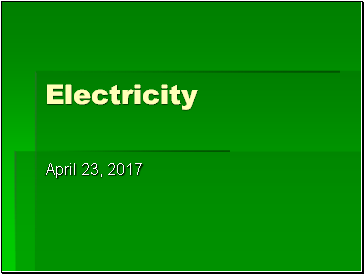Electricity

Slide 2Applications of Electrostatics

Slide 3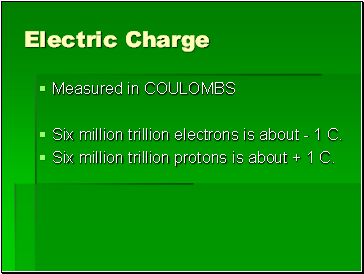## Electric Charge

Measured in COULOMBS

Six million trillion electrons is about - 1 C.

Six million trillion protons is about + 1 C.

Slide 4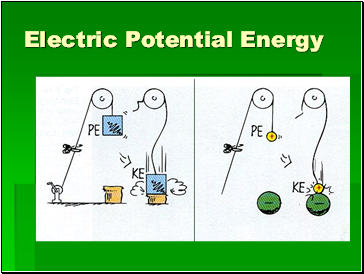## Electric Potential Energy

Slide 5Electric Potential Energy

This spring has more SPE when it is compressed

Slide 6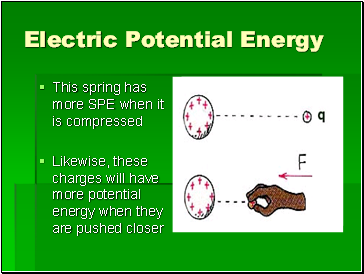Electric Potential Energy

This spring has more SPE when it is compressed

Likewise, these charges will have more potential energy when they are pushed closer

Slide 7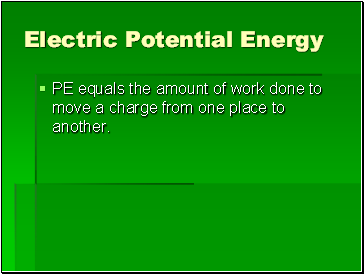Electric Potential Energy

PE equals the amount of work done to move a charge from one place to another.

Slide 8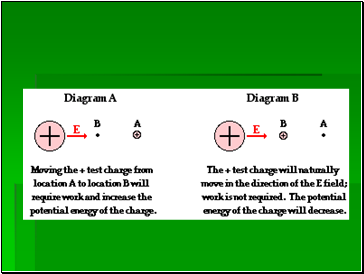Slide 9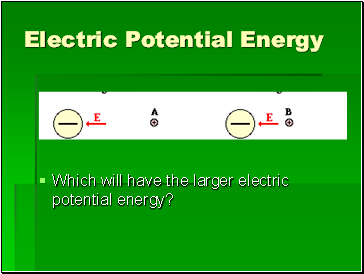Electric Potential Energy

Which will have the larger electric potential energy?

Slide 10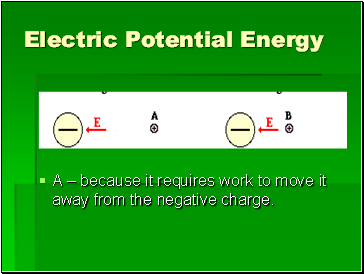Electric Potential Energy

A – because it requires work to move it away from the negative charge.

Slide 11## Electric Potential (volts)

EP = work/charge

Slide 12Electric Potential (volts)

Example: 1000 joules of work is done to move the charge q from far away to the place indicated. If q = 10 C, what is the electric potential at the new location?

Slide 13Electric Potential (volts)

Example: 1000 joules of work is done to move the charge q from far away to the place indicated. If q = 10 C, what is the electric potential at the new location?

Voltage = work/charge

V = 1000 J/10C = 100 Volts

Slide 14Electric Potential (volts)

What if the charge in the previous problem were now 100 C instead of 10? What would happen to the EP?

Slide 15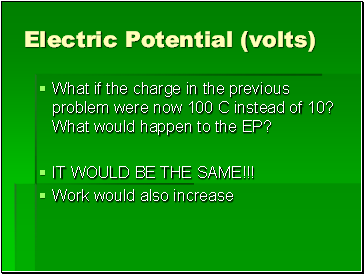Electric Potential (volts)

What if the charge in the previous problem were now 100 C instead of 10? What would happen to the EP?

Go to page:
1  2